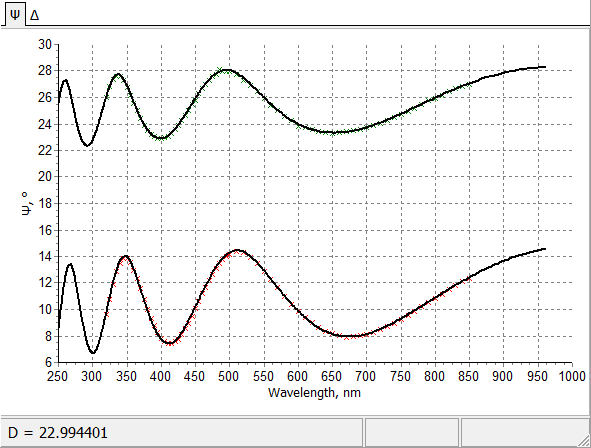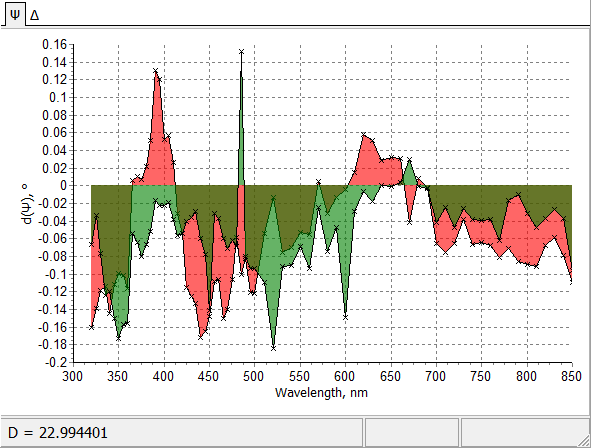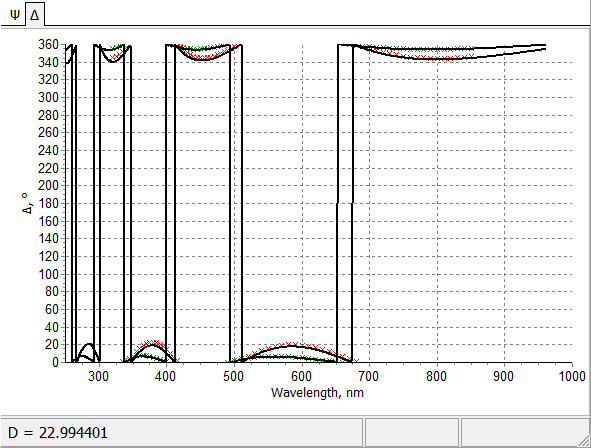### Partial Discrepancies

 Partial Discrepancies window displays the discrepancies between the loaded measurement data and the corresponding model spectral characteristics. Various partial discrepancies (for example, discrepancies for ellipsometric angles Psi and Delta) are displayed separately. This option is convenient, especially in the case of characterization on the basis of ellipsometric measurements.Intermediate fitting of measured angle Psi by model Psi angle.Partial Discrepancies calculated for Psi angle.Intermediate fitting of measured angle Delta by model Delta angle. Visually the fitting looks good. Actually it is difficult to notice deviations because of the large scale: from 0 to 360 degrees. Partial Discrepancies will help to visualize deviations.Partial Discrepancies calculated for Delta angle. Deviations between measured and model data are clearly observed. These deviations reveal deficiency of applied thin film model.